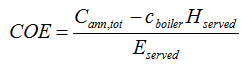# HOMER Grid 1.1

 Navigation: Glossary Levelized Cost of EnergyHOMER defines the levelized cost of energy (COE) as the average cost per kWh of useful electrical energy produced by the system.

 Type: Output Variable Units: \$/kWh Symbol: COE

To calculate the COE, HOMER divides the annualized cost of producing electricity (the total annualized cost minus the cost of serving the thermal load) by the total electric load served, using the following equation:where: Cann,tot = total annualized cost of the system [\$/yr] cboiler = boiler marginal cost [\$/kWh] Hserved = total thermal load served [kWh/yr] Eserved = total electrical load served [kWh/yr]

The second term in the numerator is the portion of the annualized cost that results from serving the thermal load. In systems, such as wind or PV, that do not serve a thermal load (Hthermal=0), this term is zero.

The COE is a convenient metric with which to compare systems, but HOMER does not rank systems based on COE.